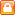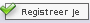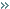Naam: Wachtwoord:Scripts:

 Scripts > PHP > Handige scripts > Directory Indexer

# Directory Indexer

Auteur: XenoX - 21 april 2005 - 17:17 - Gekeurd door: Joel - Hits: 10628 - Aantal punten: 4.85 (10 stemmen)

Dit script vervangt die saaie indexen van apache en vervangt ze door een mooiere en duidelijkere indexer.

Zet dit script in een directory en hij doet het werk.

 Code: index.php ```'] = "R0lGODlhDQAQANUAAL60urm203d3wXd3wMHB2sTG4ra5xL/K2MHO2b/N1bzM1MHP1r/O1b7N1LbKzrvN0L7Q0ZacnHh8fLq+vp6hoYqMjLu9vX1+fmRlZfz9/ez19MHIx/H49+n08uz29Ov18/f7+tS/SqGBObmXSZBqMIZoQZ2CXKmQbryqkZJ7YY14boRtbP///8fHx5eXl5OTk4mJiYODg3Z2dnBwcGlpaWBgYP///wAAAAAAAAAAAAAAAAAAAAAAAAAAAAAAAAAAACH5BAEAADYALAAAAAANABAAAAZyQJtwSCyOQiRRKBVamVpCgkBAQqkCJ4DJJRwUSp2SpwQqsWbdR2ft4YAy5+5i3Wm/47YBo3PpW+5oeQl0dnCBAxB8fRcWLHgDDYRuhl0Hin6OhwiSgF0KfBaFjw5rHxobExQVMEMGERIYNTQyMS9Ft0RBADs="; \$img['rar'] = &\$img['zip']; \$img['tar'] = &\$img['zip']; \$img['html'] = &\$img['htm']; \$img['php'] = &\$img['htm']; \$img['asp'] = &\$img['htm']; \$img['jpg'] = &\$img['img']; \$img['gif'] = &\$img['img']; \$img['png'] = &\$img['img']; \$img['bmp'] = &\$img['img']; if(IsSet(\$img[\$i])) { \$d = &\$img[\$i]; } else { \$d = &\$img['']; } header('Content-type: image/gif'); header('Content-Disposition: attachment; filename="' . \$i . '"'); echo base64_decode(\$d); exit; } function getExt(\$filename) { \$atemp = explode('.', \$filename); return strtolower(\$atemp[sizeof(\$atemp) - 1]); } function size(\$size) { \$mult = 'kB'; \$prec = 0; if(round(\$size / 1024, 0)) { \$size /= 1024; \$mult = 'kB'; \$prec = 3; } if(round(\$size / 1024, 0)) { \$size /= 1024; \$mult = 'mB'; \$prec = 3; } return (round(\$size, \$prec) . ' ' . \$mult); } function fsize(\$sfile) { return filesize(\$sfile); } function dsize(\$sdir) { \$size = 0; \$handle = opendir(\$sdir); while(false !== (\$read = readdir(\$handle))) { if(\$read <> '.' && \$read <> '..') { if(is_file(\$sdir . \$read)) { \$size += fsize(\$sdir . \$read); } elseif(is_dir(\$sdir . \$read)) { \$size += dsize(\$sdir . \$read . '/'); } } } return \$size; } if(IsSet(\$_GET['dir']) && \$_GET['dir'] <> '' && is_dir(\$_GET['dir'])) { if(\$_GET['dir'] == './') { \$dir = \$_GET['dir']; } elseif(eregi('\.\./', \$_GET['dir'])) { \$dir = './'; } else { if(substr(\$_GET['dir'], -1) == '/') { \$dir = \$_GET['dir']; } else { \$dir = \$_GET['dir'] . '/'; } } } else { \$dir = './'; } if(\$dir == './') { \$cur_dir = 'Root'; } else { \$ex = explode('/', \$dir); array_pop(\$ex); \$cur_dir = ucwords(array_pop(\$ex)); } \$handle = opendir(\$dir); while(false !== (\$read = readdir(\$handle))) { if(\$read <> '.' && \$read <> '..') { \$show[] = \$read; } } ?> Directory Indexer

'./') { \$ex = substr(\$dir, 2, -1); \$ex = explode('/', \$ex); array_pop(\$ex); \$dirs = 'Root / '; foreach(\$ex as \$nr => \$tdir) { \$link = './'; for(\$i = 0; \$i < \$nr; \$i++) { \$link .= \$ex[\$i] . '/'; } \$dirs .= '' . \$tdir . ' / '; } echo \$dirs; } ?>
Naam: Grootte: Type: Gewijzigd op:

Directory is leeg

Er staan (nog) geen mappen en/of bestanden in deze directory.

``` `'] = "R0lGODlhDQAQANUAAL60urm203d3wXd3wMHB2sTG4ra5xL/K2MHO2b/N1bzM1MHP1r/O1b7N1LbKzrvN0L7Q0ZacnHh8fLq+vp6hoYqMjLu9vX1+fmRlZfz9/ez19MHIx/H49+n08uz29Ov18/f7+tS/SqGBObmXSZBqMIZoQZ2CXKmQbryqkZJ7YY14boRtbP///8fHx5eXl5OTk4mJiYODg3Z2dnBwcGlpaWBgYP///wAAAAAAAAAAAAAAAAAAAAAAAAAAAAAAAAAAACH5BAEAADYALAAAAAANABAAAAZyQJtwSCyOQiRRKBVamVpCgkBAQqkCJ4DJJRwUSp2SpwQqsWbdR2ft4YAy5+5i3Wm/47YBo3PpW+5oeQl0dnCBAxB8fRcWLHgDDYRuhl0Hin6OhwiSgF0KfBaFjw5rHxobExQVMEMGERIYNTQyMS9Ft0RBADs="; \$img['rar'] = &\$img['zip']; \$img['tar'] = &\$img['zip']; \$img['html'] = &\$img['htm']; \$img['php'] = &\$img['htm']; \$img['asp'] = &\$img['htm']; \$img['jpg'] = &\$img['img']; \$img['gif'] = &\$img['img']; \$img['png'] = &\$img['img']; \$img['bmp'] = &\$img['img'];  if(IsSet(\$img[\$i])) { \$d = &\$img[\$i]; } else { \$d = &\$img['']; }  header('Content-type: image/gif'); header('Content-Disposition: attachment; filename="' . \$i . '"');  echo base64_decode(\$d);  exit;} function getExt(\$filename){ \$atemp = explode('.', \$filename);  return strtolower(\$atemp[sizeof(\$atemp) - 1]);} function size(\$size){ \$mult = 'kB'; \$prec = 0; if(round(\$size / 1024, 0)) { \$size /= 1024; \$mult = 'kB'; \$prec = 3; } if(round(\$size / 1024, 0)) { \$size /= 1024; \$mult = 'mB'; \$prec = 3; }  return (round(\$size, \$prec) . ' ' . \$mult);} function fsize(\$sfile){ return filesize(\$sfile);} function dsize(\$sdir){ \$size = 0;  \$handle = opendir(\$sdir); while(false !== (\$read = readdir(\$handle))) { if(\$read <> '.' && \$read <> '..') { if(is_file(\$sdir . \$read)) { \$size += fsize(\$sdir . \$read); } elseif(is_dir(\$sdir . \$read)) { \$size += dsize(\$sdir . \$read . '/'); } } }  return \$size;} if(IsSet(\$_GET['dir']) && \$_GET['dir'] <> '' && is_dir(\$_GET['dir'])){ if(\$_GET['dir'] == './') { \$dir = \$_GET['dir']; } elseif(eregi('\.\./', \$_GET['dir'])) { \$dir = './'; } else { if(substr(\$_GET['dir'], -1) == '/') { \$dir = \$_GET['dir']; } else { \$dir = \$_GET['dir'] . '/'; } }} else { \$dir = './';} if(\$dir == './'){ \$cur_dir = 'Root';}else{ \$ex = explode('/', \$dir); array_pop(\$ex); \$cur_dir = ucwords(array_pop(\$ex));} \$handle = opendir(\$dir);while(false !== (\$read = readdir(\$handle))){ if(\$read <> '.' && \$read <> '..') { \$show[] = \$read; }}?>  Directory Indexer

'./'){ \$ex = substr(\$dir, 2, -1); \$ex = explode('/', \$ex); array_pop(\$ex);  \$dirs = 'Root / '; foreach(\$ex as \$nr => \$tdir) { \$link = './'; for(\$i = 0; \$i < \$nr; \$i++) { \$link .= \$ex[\$i] . '/'; }  \$dirs .= '' . \$tdir . ' / '; }  echo \$dirs;}?>
Naam: Grootte: Type: Gewijzigd op:

Directory is leeg

Er staan (nog) geen mappen en/of bestanden in deze directory.

`Download code (.txt)Stemmen Niet ingelogd.Reacties Post een reactie Lees de reacties (19)